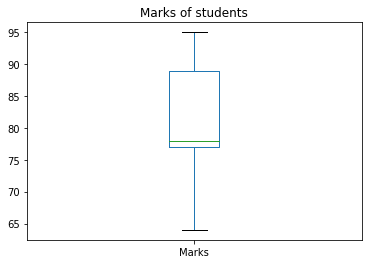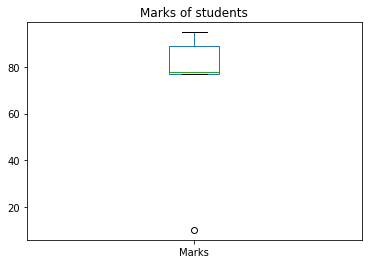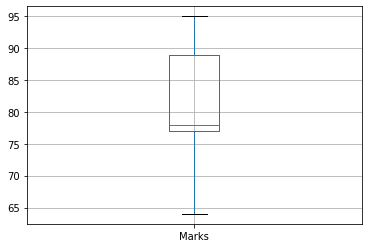# How to Create Boxplot from Pandas DataFrame?

• Last Updated : 19 Dec, 2021

Box plot is also called a Whisker plot which provides a summary of a set of data that includes minimum, first-quartile, median, third quartile, and maximum value. This Box plot is present in the matplotlib library. In the Box plot graph, the x-axis represents the data we are going to plot and the y-axis represents frequency.

## Method 1: Using DataFrame_Name[‘column_name’].plot() function

We can create a box plot on each column of a Pandas DataFrame by following the below syntax-

DataFrame_Name[‘column_name’].plot(kind=’box’, title=’title_of_plot’)

Note: We can find first quartile values, median, third quartile values using quantile method.

Syntax to find quartiles

data.quantile([0.25,0.5,0.75])

• 0.25 indicates the first quartile.
• 0.5 indicates the median value.
• 0.75 indicates the third quartile.

## Python3

 `# import necessary packages ` `import` `pandas as pd ` ` `  `data ``=` `pd.Series([``1``, ``2``, ``3``, ``4``, ``5``, ``6``]) ` ` `  `# find quartile values ` `print``(data.quantile([``0.25``, ``0.5``, ``0.75``])) `

Output

```0.25    2.25
0.50    3.50
0.75    4.75
dtype: float64```

Consider the below data to create a DataFrame and to plot a box plot on it.

Example:

Create a DataFrame using the above data and plot the Boxplot on Marks of a student. The bottom line indicates the minimum marks of a student and the top line indicates the maximum marks of a student.  Between the bottom and top, the middle 3 lines indicate 1st quartile, median, and 3rd quartile respectively.

## Python3

 `# import necessary packages ` `import` `pandas as pd ` `import` `matplotlib.pyplot as plt ` ` `  `# create a dataframe ` `data ``=` `pd.DataFrame({``'Name'``: [``'Akhil'``, ``'Nikhil'``, ``'Satyam'``, ``'Sravan'``, ``'Pavan'``], ` `                     ``'Marks'``: [``77``, ``95``, ``89``, ``78``, ``64``], ` `                     ``'Credits'``: [``8``, ``10``, ``9``, ``8``, ``7``]}) ` ` `  `# box plot ` `data[``'Marks'``].plot(kind``=``'box'``, title``=``'Marks of students'``) ` `plt.show() `

Output:Example:

In this example, the minimum mark of the student is 10 which is very small and far from other marks (data points). So it is indicated as o at the bottom which represents an outlier.  If any of the data points in the data is much larger or smaller compared to other values then the following plot will be generated.

## Python3

 `# import necessary packages ` `import` `pandas as pd ` `import` `matplotlib.pyplot as plt ` ` `  `# create a dataframe ` `data ``=` `pd.DataFrame({``'Name'``: [``'Akhil'``, ``'Nikhil'``, ``'Satyam'``, ``'Sravan'``, ``'Pavan'``], ` `                     ``'Marks'``: [``77``, ``95``, ``89``, ``78``, ``10``], ` `                     ``'Credits'``: [``8``, ``10``, ``9``, ``8``, ``0``]}) ` ` `  `# outlier box plot ` `data[``'Marks'``].plot(kind``=``'box'``, title``=``'Marks of students'``) ` `plt.show() `

Output:## Method 2: Using pandas.DataFrame.boxplot() function

We can also use pandas.DataFrame.boxplot to draw the box plot for respective columns in a DataFrame.

Syntax

DataFrameName.boxplot(column=’column_name’,grid=True/False)

grid indicates grid lines in a graph. It is an optional parameter, if not specified it will be considered as true.

Example:

Here we plotted the boxplot using the boxplot method instead of using the plot method and specifying its kind. As we did not specify the grid argument as a parameter in the boxplot method, it will consider the default value i.e. True.

## Python3

 `# import necessary packages ` `import` `pandas as pd ` ` `  `# create a dataframe ` `data ``=` `pd.DataFrame({``'Name'``: [``'Akhil'``, ``'Nikhil'``, ``'Satyam'``, ``'Sravan'``, ``'Pavan'``], ` `                     ``'Marks'``: [``77``, ``95``, ``89``, ``78``, ``64``], ` `                     ``'Credits'``: [``8``, ``10``, ``9``, ``8``, ``7``]}) ` ` `  `# box plot for marks column ` `data.boxplot(column``=``'Marks'``) `

Output:My Personal Notes arrow_drop_up
Recommended Articles
Page :